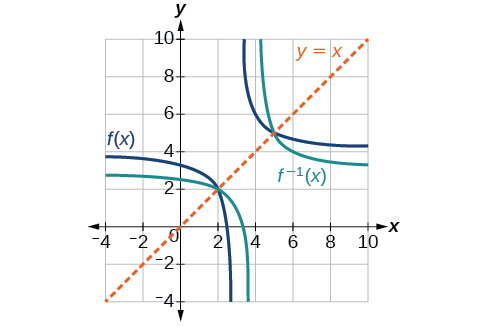3.7 Inverse functions  (Page 6/9)

 Page 6 / 9

Draw graphs of the functions and from [link] .Is there any function that is equal to its own inverse?

Yes. If $\text{\hspace{0.17em}}f={f}^{-1},\text{\hspace{0.17em}}$ then $\text{\hspace{0.17em}}f\left(f\left(x\right)\right)=x,\text{\hspace{0.17em}}$ and we can think of several functions that have this property. The identity function does, and so does the reciprocal function, because

$\frac{1}{\frac{1}{x}}=x$

Any function $\text{\hspace{0.17em}}f\left(x\right)=c-x,\text{\hspace{0.17em}}$ where $\text{\hspace{0.17em}}c\text{\hspace{0.17em}}$ is a constant, is also equal to its own inverse.

Access these online resources for additional instruction and practice with inverse functions.

Visit this website for additional practice questions from Learningpod.

Key concepts

• If $\text{\hspace{0.17em}}g\left(x\right)\text{\hspace{0.17em}}$ is the inverse of $\text{\hspace{0.17em}}f\left(x\right),\text{\hspace{0.17em}}$ then $\text{\hspace{0.17em}}g\left(f\left(x\right)\right)=f\left(g\left(x\right)\right)=x.\text{\hspace{0.17em}}$ See [link] , [link] , and [link] .
• Only some of the toolkit functions have an inverse. See [link] .
• For a function to have an inverse, it must be one-to-one (pass the horizontal line test).
• A function that is not one-to-one over its entire domain may be one-to-one on part of its domain.
• For a tabular function, exchange the input and output rows to obtain the inverse. See [link] .
• The inverse of a function can be determined at specific points on its graph. See [link] .
• To find the inverse of a formula, solve the equation $\text{\hspace{0.17em}}y=f\left(x\right)\text{\hspace{0.17em}}$ for $\text{\hspace{0.17em}}x\text{\hspace{0.17em}}$ as a function of $\text{\hspace{0.17em}}y.\text{\hspace{0.17em}}$ Then exchange the labels $\text{\hspace{0.17em}}x\text{\hspace{0.17em}}$ and $\text{\hspace{0.17em}}\text{\hspace{0.17em}}y.\text{\hspace{0.17em}}\text{\hspace{0.17em}}$ See [link] , [link] , and [link] .
• The graph of an inverse function is the reflection of the graph of the original function across the line $\text{\hspace{0.17em}}y=x.\text{\hspace{0.17em}}$ See [link] .

Verbal

Describe why the horizontal line test is an effective way to determine whether a function is one-to-one?

Each output of a function must have exactly one output for the function to be one-to-one. If any horizontal line crosses the graph of a function more than once, that means that $\text{\hspace{0.17em}}y$ -values repeat and the function is not one-to-one. If no horizontal line crosses the graph of the function more than once, then no $\text{\hspace{0.17em}}y$ -values repeat and the function is one-to-one.

Why do we restrict the domain of the function $\text{\hspace{0.17em}}f\left(x\right)={x}^{2}\text{\hspace{0.17em}}$ to find the function’s inverse?

Can a function be its own inverse? Explain.

Yes. For example, $\text{\hspace{0.17em}}f\left(x\right)=\frac{1}{x}\text{\hspace{0.17em}}$ is its own inverse.

Are one-to-one functions either always increasing or always decreasing? Why or why not?

How do you find the inverse of a function algebraically?

Given a function $\text{\hspace{0.17em}}y=f\left(x\right),\text{\hspace{0.17em}}$ solve for $\text{\hspace{0.17em}}x\text{\hspace{0.17em}}$ in terms of $\text{\hspace{0.17em}}y.\text{\hspace{0.17em}}$ Interchange the $\text{\hspace{0.17em}}x\text{\hspace{0.17em}}$ and $\text{\hspace{0.17em}}y.\text{\hspace{0.17em}}$ Solve the new equation for $\text{\hspace{0.17em}}y.\text{\hspace{0.17em}}$ The expression for $\text{\hspace{0.17em}}y\text{\hspace{0.17em}}$ is the inverse, $\text{\hspace{0.17em}}y={f}^{-1}\left(x\right).$

Algebraic

Show that the function $\text{\hspace{0.17em}}f\left(x\right)=a-x\text{\hspace{0.17em}}$ is its own inverse for all real numbers $\text{\hspace{0.17em}}a.\text{\hspace{0.17em}}$

For the following exercises, find $\text{\hspace{0.17em}}{f}^{-1}\left(x\right)\text{\hspace{0.17em}}$ for each function.

$f\left(x\right)=x+3$

${f}^{-1}\left(x\right)=x-3$

$f\left(x\right)=x+5$

$f\left(x\right)=2-x$

${f}^{-1}\left(x\right)=2-x$

$f\left(x\right)=3-x$

$f\left(x\right)=\frac{x}{x+2}$

${f}^{-1}\left(x\right)=\frac{-2x}{x-1}$

$f\left(x\right)=\frac{2x+3}{5x+4}$

For the following exercises, find a domain on which each function $\text{\hspace{0.17em}}f\text{\hspace{0.17em}}$ is one-to-one and non-decreasing. Write the domain in interval notation. Then find the inverse of $\text{\hspace{0.17em}}f\text{\hspace{0.17em}}$ restricted to that domain.

$f\left(x\right)={\left(x+7\right)}^{2}$

domain of $f\left(x\right):\text{\hspace{0.17em}}\left[-7,\infty \right);\text{\hspace{0.17em}}{f}^{-1}\left(x\right)=\sqrt{x}-7$

$f\left(x\right)={\left(x-6\right)}^{2}$

$f\left(x\right)={x}^{2}-5$

domain of $\text{\hspace{0.17em}}f\left(x\right):\text{\hspace{0.17em}}\left[0,\infty \right);\text{\hspace{0.17em}}{f}^{-1}\left(x\right)=\sqrt{x+5}$

Given $\text{\hspace{0.17em}}f\left(x\right)=\frac{x}{2+x}\text{\hspace{0.17em}}$ and $\text{\hspace{0.17em}}g\left(x\right)=\frac{2x}{1-x}:$

1. Find $\text{\hspace{0.17em}}f\left(g\left(x\right)\right)\text{\hspace{0.17em}}$ and $\text{\hspace{0.17em}}g\left(f\left(x\right)\right).$
2. What does the answer tell us about the relationship between $\text{\hspace{0.17em}}f\left(x\right)\text{\hspace{0.17em}}$ and $\text{\hspace{0.17em}}g\left(x\right)?$

a. and $\text{\hspace{0.17em}}g\left(f\left(x\right)\right)=x.\text{\hspace{0.17em}}$ b. This tells us that $\text{\hspace{0.17em}}f\text{\hspace{0.17em}}$ and $\text{\hspace{0.17em}}g\text{\hspace{0.17em}}$ are inverse functions

The sequence is {1,-1,1-1.....} has
how can we solve this problem
Sin(A+B) = sinBcosA+cosBsinA
Prove it
Eseka
Eseka
hi
Joel
June needs 45 gallons of punch. 2 different coolers. Bigger cooler is 5 times as large as smaller cooler. How many gallons in each cooler?
7.5 and 37.5
Nando
find the sum of 28th term of the AP 3+10+17+---------
I think you should say "28 terms" instead of "28th term"
Vedant
the 28th term is 175
Nando
192
Kenneth
if sequence sn is a such that sn>0 for all n and lim sn=0than prove that lim (s1 s2............ sn) ke hole power n =n
write down the polynomial function with root 1/3,2,-3 with solution
if A and B are subspaces of V prove that (A+B)/B=A/(A-B)
write down the value of each of the following in surd form a)cos(-65°) b)sin(-180°)c)tan(225°)d)tan(135°)
Prove that (sinA/1-cosA - 1-cosA/sinA) (cosA/1-sinA - 1-sinA/cosA) = 4
what is the answer to dividing negative index
In a triangle ABC prove that. (b+c)cosA+(c+a)cosB+(a+b)cisC=a+b+c.
give me the waec 2019 questions
the polar co-ordinate of the point (-1, -1)ByByBy Lakeima RobertsByBy Dravida Mahadeo-J...